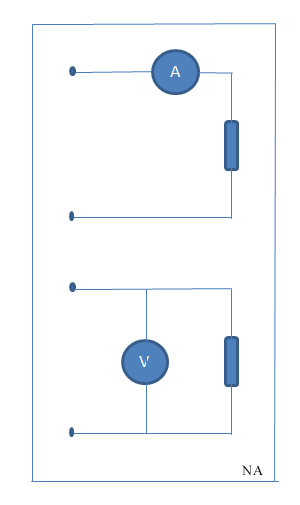What is the correct way to connect a voltmeter to a circuit? A. In series with the circuit B. In parallel with the circuit C. In quadrature with the circuit…

# Technician: Connecting a Voltmeter

## What is the correct way to connect a voltmeter to a circuit?

A. In series with the circuit
B. In parallel with the circuit
C. In quadrature with the circuit
D. In phase with the circuit

d’

Well, first off lets just get rid of C. and D. Neither “in quadrature” or “in phase” really make any sense at all.

So do we connect the probes of our voltmeter in parallel or in series to a circuit? We need to make sure we understand what each of those terms mean.

“In parallel” means we connect each probe to either end of the circuit in question.  You’ll hear the term “voltage across” a circuit, battery, or component quite a bit.  This is an easy way to remember parallel.  For example, you measure the voltage of a battery by connecting the voltmeter “across” the terminals, or simply one at each.  It would look something like the image below, where the voltmeter is “V.”

Series, on the other hand, involves inserting your meter into the circuit as part of the “chain” or “path” of the circuit, and looks something like the image below, where the ammeter is “A.”Attempting to measure voltage in this manner will  just not work.  Instead, you measure current, or amps this way.  By putting your ammeter “in the path” you can measure the amount of charge flowing through the circuit.

So, the correct answer is, B. In parallel with the circuit.

[T7D02]